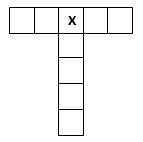# X Marks The SquarePlace integers from 1 to 9 (once each) into the 9 squares above, such that the horizontal row sums to 30 and the vertical column sums to 22.

What number would you place in square X?

×